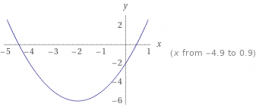# 11990 perimeter RT

A right triangle has integer side lengths and a perimeter of 11990. In addition, we know that one of its perpendiculars has a prime number length. Find its length.

a =  109

### Step-by-step explanation:Did you find an error or inaccuracy? Feel free to write us. Thank you!

Tips for related online calculators
Are you looking for help with calculating roots of a quadratic equation?
Do you have a system of equations and looking for calculator system of linear equations?
Do you solve Diofant problems and looking for a calculator of Diofant integer equations?